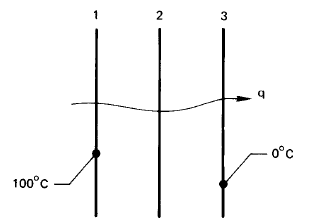# Series of black plates

LCSphysicist
Homework Statement:
Print below
Relevant Equations:
\nFirst i computed the rate of energy wrt time of the second plate:
$$dq_{2}/dt = A \sigma ((373)^4/2 + (273)^4/2 - T_{2}^4)$$
Equaliting it to zero we get the answer. But i am not sure why do we need to equality it to zero.
The q arrow on the figure suggest me that it is conservation of energy (radiation flux), but i don't get why.
The black body has transmission coefficient ##\tau = 0##, so i don't understand why the flux of radiation need to be equal. The body could pretty well absorb the energy coming from 1 and emite an energy to 3 totally different, couldn't?

•